# Direct relationship - math word problems

#### Number of problems found: 296

• Angles ratioIn a triangle ABC true relationship c is less than b and b is less than a. Internal angles of the triangle are in the ratio 5:4:9. The size of the internal angle beta is:
• ExpressionIf it is true that ? is:
• Michal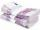Michal has 2.90 per hour. How much does he earn during the week of the summer job?
• Six studentsTwo pupils painted the class in four hours. How long will it take for six pupils?
• A bucketA bucket has 4 liters of water in it when it is 2/5 full. How much can it hold?
• Map 3Map scale is M = 1: 25000 . Two cottages which are shown on the map are actually 15 km away. What is its distance on the map?
• Simple interest 3Find the simple interest if 11928 USD at 2% for 10 weeks.
• Package Deal - what to choose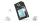The parents decided to purchase a mobile phone to their son. They choose from menu: ? Which offer is best?
• Report 2A School reports students to teacher ratio of 6:1. If there are 45 teachers in the School, how many students are there?
• Slope RR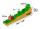A line has a rise of 2 and a run of 11. What is the slope?
• DonutsFind how many donuts each student will receive if you share 126 donuts in a ratio of 1:5:8
• Cyclist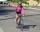Cyclist drives 5 km in half an hour, how long will drive in 3 hours?
• Ratio6 numbers are in the ratio 1:5:1:5:5:5. Their sum is 242. What are the numbers?
• Euros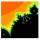Michal, Peter, John and Lenka got together 2,400 euros. They share an amount in ratio 2:6:4:3. How many got each of them?
• Motor oil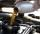30 liters of oil cost 28.80 Euros. How much cost a liter?
• Simple interest 2Find the simple interest if 13905 USD at 4.5% for 6 months.
• RunnersFor three runners (on the first to third place) is prepared 30 chocolate that they be distributed in the ratio of 3 : 2 : 1 How much chocolate will get everyone?
• Moneys in triple ratioMilan, John and Lili have a total 344 euros. Their amounts are in the ratio 1:2:5. Determine how much each of them has?
• Pump680 liters of water were pumped in 8 minutes. How many liters was spent in 56 minutes?
• Divide moneyDivide 1200 USD at a ratio of 1:2:3:4:5:6:9:10

Do you have an interesting mathematical word problem that you can't solve it? Submit a math problem, and we can try to solve it.

We will send a solution to your e-mail address. Solved examples are also published here. Please enter the e-mail correctly and check whether you don't have a full mailbox.

Please do not submit problems from current active competitions such as Mathematical Olympiad, correspondence seminars etc...